Paper Folding Quiz I, Non Verbal Reasoning

# Paper Folding Quiz I, Non Verbal Reasoning - Mechanical Engineering

Test Description

## 20 Questions MCQ Test General Aptitude for GATE - Paper Folding Quiz I, Non Verbal Reasoning

Paper Folding Quiz I, Non Verbal Reasoning for Mechanical Engineering 2023 is part of General Aptitude for GATE preparation. The Paper Folding Quiz I, Non Verbal Reasoning questions and answers have been prepared according to the Mechanical Engineering exam syllabus.The Paper Folding Quiz I, Non Verbal Reasoning MCQs are made for Mechanical Engineering 2023 Exam. Find important definitions, questions, notes, meanings, examples, exercises, MCQs and online tests for Paper Folding Quiz I, Non Verbal Reasoning below.
Solutions of Paper Folding Quiz I, Non Verbal Reasoning questions in English are available as part of our General Aptitude for GATE for Mechanical Engineering & Paper Folding Quiz I, Non Verbal Reasoning solutions in Hindi for General Aptitude for GATE course. Download more important topics, notes, lectures and mock test series for Mechanical Engineering Exam by signing up for free. Attempt Paper Folding Quiz I, Non Verbal Reasoning | 20 questions in 40 minutes | Mock test for Mechanical Engineering preparation | Free important questions MCQ to study General Aptitude for GATE for Mechanical Engineering Exam | Download free PDF with solutions
 1 Crore+ students have signed up on EduRev. Have you?
Paper Folding Quiz I, Non Verbal Reasoning - Question 1

### Directions to Solve In each of the following problems, a square transparent sheet (X) with a pattern is given. Figure out from amongst the four alternatives as to how the patter would appear when the transparent sheet is folded at the dotted line. Question - Find out from amongst the four alternatives as to how the pattern would appear when the transparent sheet is folded at the dotted line.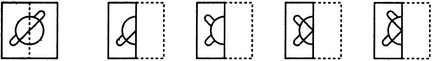(X)                     (1)                 (2)                 (3)                 (4)

Paper Folding Quiz I, Non Verbal Reasoning - Question 2

### Directions to Solve In each of the following problems, a square transparent sheet (X) with a pattern is given. Figure out from amongst the four alternatives as to how the patter would appear when the transparent sheet is folded at the dotted line. Question - Find out from amongst the four alternatives as to how the pattern would appear when the transparent sheet is folded at the dotted line.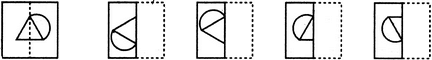(X)                     (1)                 (2)                 (3)                 (4)

Paper Folding Quiz I, Non Verbal Reasoning - Question 3

### Directions to Solve In each of the following problems, a square transparent sheet (X) with a pattern is given. Figure out from amongst the four alternatives as to how the patter would appear when the transparent sheet is folded at the dotted line. Question - Find out from amongst the four alternatives as to how the pattern would appear when the transparent sheet is folded at the dotted line.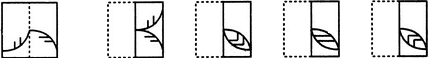(X)                     (1)                 (2)                 (3)                 (4)

Paper Folding Quiz I, Non Verbal Reasoning - Question 4

In each of the following problems, a square transparent sheet (X) with a pattern is given. Figure out from amongst the four alternatives as to how the patter would appear when the transparent sheet is folded at the dotted line.

Question -

Find out from amongst the four alternatives as to how the pattern would appear when the transparent sheet is folded at the dotted line.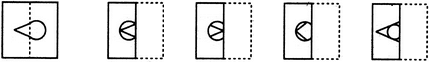(X)                     (1)                 (2)                 (3)                 (4)
Paper Folding Quiz I, Non Verbal Reasoning - Question 5

In each of the following problems, a square transparent sheet (X) with a pattern is given. Figure out from amongst the four alternatives as to how the patter would appear when the transparent sheet is folded at the dotted line.

Question -

Find out from amongst the four alternatives as to how the pattern would appear when the transparent sheet is folded at the dotted line.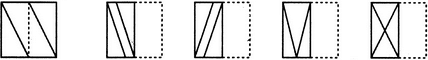(X)                     (1)                 (2)                 (3)                 (4)
Paper Folding Quiz I, Non Verbal Reasoning - Question 6

In each of the following problems, a square transparent sheet (X) with a pattern is given. Figure out from amongst the four alternatives as to how the patter would appear when the transparent sheet is folded at the dotted line.

Question -

Find out from amongst the four alternatives as to how the pattern would appear when the transparent sheet is folded at the dotted line.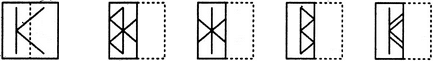(X)                     (1)                 (2)                 (3)                 (4)
Paper Folding Quiz I, Non Verbal Reasoning - Question 7

In each of the following problems, a square transparent sheet (X) with a pattern is given. Figure out from amongst the four alternatives as to how the patter would appear when the transparent sheet is folded at the dotted line.

Question -

Find out from amongst the four alternatives as to how the pattern would appear when the transparent sheet is folded at the dotted line.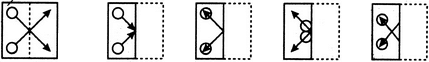(X)                     (1)                 (2)                 (3)                 (4)
Paper Folding Quiz I, Non Verbal Reasoning - Question 8

In each of the following problems, a square transparent sheet (X) with a pattern is given. Figure out from amongst the four alternatives as to how the patter would appear when the transparent sheet is folded at the dotted line.

Question -

Find out from amongst the four alternatives as to how the pattern would appear when the transparent sheet is folded at the dotted line.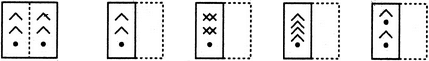(X)                     (1)                 (2)                 (3)                 (4)
Paper Folding Quiz I, Non Verbal Reasoning - Question 9

In each of the following problems, a square transparent sheet (X) with a pattern is given. Figure out from amongst the four alternatives as to how the patter would appear when the transparent sheet is folded at the dotted line.

Question -

Find out from amongst the four alternatives as to how the pattern would appear when the transparent sheet is folded at the dotted line.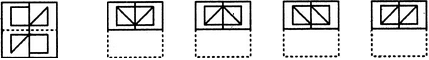(X)                     (1)                 (2)                 (3)                 (4)
Paper Folding Quiz I, Non Verbal Reasoning - Question 10

In each of the following problems, a square transparent sheet (X) with a pattern is given. Figure out from amongst the four alternatives as to how the patter would appear when the transparent sheet is folded at the dotted line.

Question -

Find out from amongst the four alternatives as to how the pattern would appear when the transparent sheet is folded at the dotted line.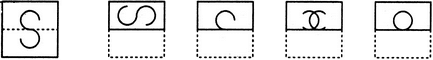(X)                     (1)                 (2)                 (3)                 (4)
Paper Folding Quiz I, Non Verbal Reasoning - Question 11

In each of the following problems, a square transparent sheet (X) with a pattern is given. Figure out from amongst the four alternatives as to how the patter would appear when the transparent sheet is folded at the dotted line.

Question -

Find out from amongst the four alternatives as to how the pattern would appear when the transparent sheet is folded at the dotted line.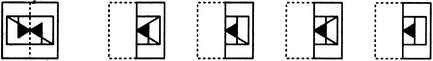(X)                     (1)                 (2)                 (3)                 (4)
Paper Folding Quiz I, Non Verbal Reasoning - Question 12

In each of the following problems, a square transparent sheet (X) with a pattern is given. Figure out from amongst the four alternatives as to how the patter would appear when the transparent sheet is folded at the dotted line.

Question -

Find out from amongst the four alternatives as to how the pattern would appear when the transparent sheet is folded at the dotted line.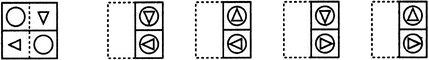(X)                     (1)                 (2)                 (3)                 (4)
Paper Folding Quiz I, Non Verbal Reasoning - Question 13

In each of the following problems, a square transparent sheet (X) with a pattern is given. Figure out from amongst the four alternatives as to how the patter would appear when the transparent sheet is folded at the dotted line.

Question -

Find out from amongst the four alternatives as to how the pattern would appear when the transparent sheet is folded at the dotted line.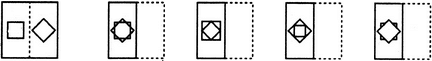(X)                     (1)                 (2)                 (3)                 (4)
Paper Folding Quiz I, Non Verbal Reasoning - Question 14

In each of the following problems, a square transparent sheet (X) with a pattern is given. Figure out from amongst the four alternatives as to how the patter would appear when the transparent sheet is folded at the dotted line.

Question -

Find out from amongst the four alternatives as to how the pattern would appear when the transparent sheet is folded at the dotted line.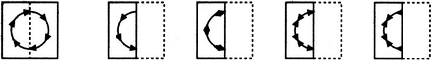(X)                     (1)                 (2)                 (3)                 (4)
Paper Folding Quiz I, Non Verbal Reasoning - Question 15

In each of the following problems, a square transparent sheet (X) with a pattern is given. Figure out from amongst the four alternatives as to how the patter would appear when the transparent sheet is folded at the dotted line.

Question -

Find out from amongst the four alternatives as to how the pattern would appear when the transparent sheet is folded at the dotted line.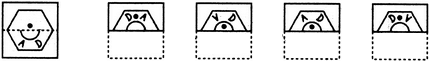(X)                     (1)                 (2)                 (3)                 (4)
Paper Folding Quiz I, Non Verbal Reasoning - Question 16

In each of the following problems, a square transparent sheet (X) with a pattern is given. Figure out from amongst the four alternatives as to how the patter would appear when the transparent sheet is folded at the dotted line.

Question -

Find out from amongst the four alternatives as to how the pattern would appear when the transparent sheet is folded at the dotted line.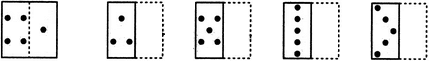(X)                     (1)                 (2)                 (3)                 (4)
Paper Folding Quiz I, Non Verbal Reasoning - Question 17

In each of the following problems, a square transparent sheet (X) with a pattern is given. Figure out from amongst the four alternatives as to how the patter would appear when the transparent sheet is folded at the dotted line.

Question -

Find out from amongst the four alternatives as to how the pattern would appear when the transparent sheet is folded at the dotted line.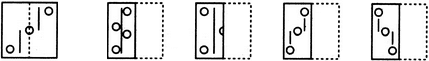(X)                     (1)                 (2)                 (3)                 (4)
Paper Folding Quiz I, Non Verbal Reasoning - Question 18

In each of the following problems, a square transparent sheet (X) with a pattern is given. Figure out from amongst the four alternatives as to how the patter would appear when the transparent sheet is folded at the dotted line.

Question -

Find out from amongst the four alternatives as to how the pattern would appear when the transparent sheet is folded at the dotted line.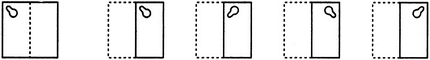(X)                     (1)                 (2)                 (3)                 (4)
Paper Folding Quiz I, Non Verbal Reasoning - Question 19

In each of the following problems, a square transparent sheet (X) with a pattern is given. Figure out from amongst the four alternatives as to how the patter would appear when the transparent sheet is folded at the dotted line.

Question -

Find out from amongst the four alternatives as to how the pattern would appear when the transparent sheet is folded at the dotted line.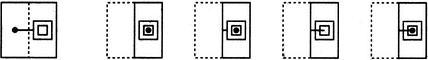(X)                     (1)                 (2)                 (3)                 (4)
Paper Folding Quiz I, Non Verbal Reasoning - Question 20

Directions to Solve

In each of the following problems, a square transparent sheet (X) with a pattern is given. Figure out from amongst the four alternatives as to how the patter would appear when the transparent sheet is folded at the dotted line.

Question -

Find out from amongst the four alternatives as to how the pattern would appear when the transparent sheet is folded at the dotted line.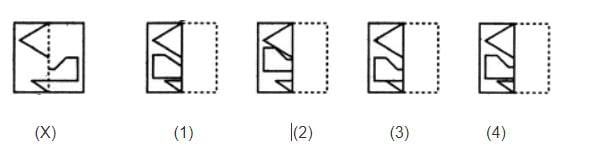## General Aptitude for GATE

84 videos|84 docs|108 tests
Information about Paper Folding Quiz I, Non Verbal Reasoning Page
In this test you can find the Exam questions for Paper Folding Quiz I, Non Verbal Reasoning solved & explained in the simplest way possible. Besides giving Questions and answers for Paper Folding Quiz I, Non Verbal Reasoning, EduRev gives you an ample number of Online tests for practice

## General Aptitude for GATE

84 videos|84 docs|108 tests